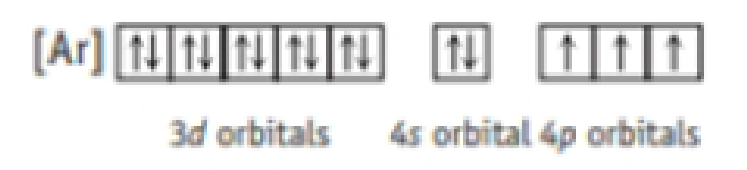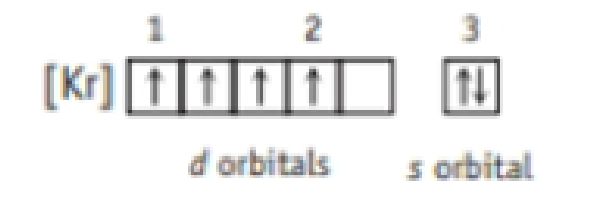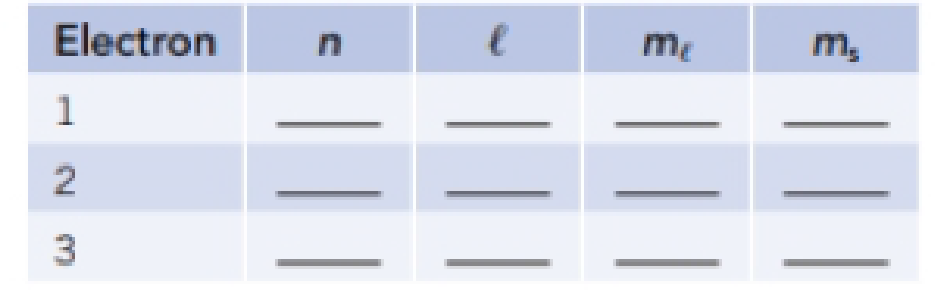# Answer the questions below concerning ground state electron configurations. (a) What element has the electron configuration [Ar]3 d 6 4 s 2 ? (b) What element has a 2+ ion with the configuration [Ar]3 d 5 ? Is the ion paramagnetic or diamagnetic? (c) Mow many unpaired electrons are in a Ni 2+ ion? (d) The configuration for an element is given here. What is the identity of the element? Is a sample of the element paramagnetic or diamagnetic? How many unpaired electrons does a 3− ion of this element have? (e) What element has the following electron configuration? Write a complete set of quantum numbers for electrons 1-3.### Chemistry & Chemical Reactivity

9th Edition
John C. Kotz + 3 others
Publisher: Cengage Learning
ISBN: 9781133949640

#### Solutions

Chapter
Section### Chemistry & Chemical Reactivity

9th Edition
John C. Kotz + 3 others
Publisher: Cengage Learning
ISBN: 9781133949640
Chapter 7, Problem 60GQ
Textbook Problem
19 views

## Answer the questions below concerning ground state electron configurations. (a) What element has the electron configuration [Ar]3d64s2? (b) What element has a 2+ ion with the configuration [Ar]3d5? Is the ion paramagnetic or diamagnetic? (c) Mow many unpaired electrons are in a Ni2+ ion? (d) The configuration for an element is given here.What is the identity of the element? Is a sample of the element paramagnetic or diamagnetic? How many unpaired electrons does a 3− ion of this element have? (e) What element has the following electron configuration? Write a complete set of quantum numbers for electrons 1-3.a)

Interpretation Introduction

Interpretation:

The element that has electron configuration [Ar]3d54s2 has to be predicted.

Concept Introduction:

Electronic configuration: The electronic configuration is the distribution of electrons of an given molecule or respective atoms in atomic or molecular orbital’s. The important there rules for electronic configuration given below.

Aufbau principle: This rule statues that ground state of an atom or ions electrons fill atomic orbitals of the lowest available energy levels before occupying higher levels. If consider the 1s shell is filled the 2s subshell is occupied.

Paramagnetic: The Paramagnetic properties are due to the presence of some unpaired electrons, and from the realignment of the electron paths caused by the external magnetic field.

Diamagnetic properties: In diamagnetic materials all the electron are paired so there is no permanent net magnetic moment per atom.

Quantum numbers: These terms are explained for the distribution of electron density in an atom. They are derived from the mathematical solution of Schrodinger’s equation for the hydrogen atom.  The types of quantum numbers are the principal quantum number (n), the angular momentum quantum number (l), the magnetic quantum number (ml) and the electron spin quantum number (ms). Each atomic orbital in an atom is categorized by a unique set of the quantum numbers.

### Explanation of Solution

The element that has the given electronic configuration is, IRON.

The electronic configuration of iron is [Ar]3d64s2

AtomicnumberofIron (Fe)=26spdfwith noble gas notation=[Ar]3d64s2Orbitalboxnotation       =  [Ar]

b)

Interpretation Introduction

Interpretation:

The element ion that has configuration [Ar]3d5 has to be predicted.

Concept Introduction:

Electronic configuration: The electronic configuration is the distribution of electrons of an given molecule or respective atoms in atomic or molecular orbital’s. The important there rules for electronic configuration given below.

Aufbau principle: This rule statues that ground state of an atom or ions electrons fill atomic orbitals of the lowest available energy levels before occupying higher levels. If consider the 1s shell is filled the 2s subshell is occupied.

Paramagnetic: The Paramagnetic properties are due to the presence of some unpaired electrons, and from the realignment of the electron paths caused by the external magnetic field.

Diamagnetic properties: In diamagnetic materials all the electron are paired so there is no permanent net magnetic moment per atom.

Quantum numbers: These terms are explained for the distribution of electron density in an atom. They are derived from the mathematical solution of Schrodinger’s equation for the hydrogen atom.  The types of quantum numbers are the principal quantum number (n), the angular momentum quantum number (l), the magnetic quantum number (ml) and the electron spin quantum number (ms). Each atomic orbital in an atom is categorized by a unique set of the quantum numbers.

c)

Interpretation Introduction

Interpretation:

The number of unpaired electrons present in Ni2+ has to be predicted.

Concept Introduction:

Electronic configuration: The electronic configuration is the distribution of electrons of an given molecule or respective atoms in atomic or molecular orbital’s. The important there rules for electronic configuration given below.

Aufbau principle: This rule statues that ground state of an atom or ions electrons fill atomic orbitals of the lowest available energy levels before occupying higher levels. If consider the 1s shell is filled the 2s subshell is occupied.

Paramagnetic: The Paramagnetic properties are due to the presence of some unpaired electrons, and from the realignment of the electron paths caused by the external magnetic field.

Diamagnetic properties: In diamagnetic materials all the electron are paired so there is no permanent net magnetic moment per atom.

Quantum numbers: These terms are explained for the distribution of electron density in an atom. They are derived from the mathematical solution of Schrodinger’s equation for the hydrogen atom.  The types of quantum numbers are the principal quantum number (n), the angular momentum quantum number (l), the magnetic quantum number (ml) and the electron spin quantum number (ms). Each atomic orbital in an atom is categorized by a unique set of the quantum numbers.

d)

Interpretation Introduction

Interpretation:

The identity of element, paramagnetic or diamagnetic; and number of unpaired  electrons does a 3- ion of this element contains has to be predicted.

Concept Introduction:

Electronic configuration: The electronic configuration is the distribution of electrons of an given molecule or respective atoms in atomic or molecular orbital’s. The important there rules for electronic configuration given below.

Aufbau principle: This rule statues that ground state of an atom or ions electrons fill atomic orbitals of the lowest available energy levels before occupying higher levels. If consider the 1s shell is filled the 2s subshell is occupied.

Paramagnetic: The Paramagnetic properties are due to the presence of some unpaired electrons, and from the realignment of the electron paths caused by the external magnetic field.

Diamagnetic properties: In diamagnetic materials all the electron are paired so there is no permanent net magnetic moment per atom.

Quantum numbers: These terms are explained for the distribution of electron density in an atom. They are derived from the mathematical solution of Schrodinger’s equation for the hydrogen atom.  The types of quantum numbers are the principal quantum number (n), the angular momentum quantum number (l), the magnetic quantum number (ml) and the electron spin quantum number (ms). Each atomic orbital in an atom is categorized by a unique set of the quantum numbers.

e)

Interpretation Introduction

Interpretation:

The periodic table clearly explained the electronic configuration, ionic properties, electro negativity and magnetic properties for each (s, p, d, f) block element, the different terms are explained given the statement. So in this problem correct element, paramagnetic properties and quantum number are should be derived given the orbital notation method.

Concept Introduction:

Electronic configuration: The electronic configuration is the distribution of electrons of an given molecule or respective atoms in atomic or molecular orbital’s. The important there rules for electronic configuration given below.

Aufbau principle: This rule statues that ground state of an atom or ions electrons fill atomic orbitals of the lowest available energy levels before occupying higher levels. If consider the 1s shell is filled the 2s subshell is occupied.

Quantum numbers: These terms are explained for the distribution of electron density in an atom. They are derived from the mathematical solution of Schrodinger’s equation for the hydrogen atom.  The types of quantum numbers are the principal quantum number (n), the angular momentum quantum number (l), the magnetic quantum number (ml) and the electron spin quantum number (ms). Each atomic orbital in an atom is categorized by a unique set of the quantum numbers.

### Still sussing out bartleby?

Check out a sample textbook solution.

See a sample solution

#### The Solution to Your Study Problems

Bartleby provides explanations to thousands of textbook problems written by our experts, many with advanced degrees!

Get Started

Find more solutions based on key concepts
What are the features of a healthy high-fat diet?

Understanding Nutrition (MindTap Course List)

Amino adds are linked together to form a protein strand by peptide bonds. essential amino acid bonds. side chai...

Nutrition: Concepts and Controversies - Standalone book (MindTap Course List)

In the following diagram, designate each daughter cell as diploid (2n) or haploid (n).

Human Heredity: Principles and Issues (MindTap Course List)

How does a covalent bond form?

Biology: The Dynamic Science (MindTap Course List)

Is the light chain of an immunoglobulin the same as the V region?

Introduction to General, Organic and Biochemistry

Two spheres having masses M and 2M and radii R and 3R, respectively, are simultaneously released from rest when...

Physics for Scientists and Engineers, Technology Update (No access codes included)

How do some variable stars confirm that stars have evolved?

Foundations of Astronomy (MindTap Course List)

___ attach mosses to soil. a. Rhizoids b. Rhizomes c. Roots d. Strobili

Biology: The Unity and Diversity of Life (MindTap Course List)